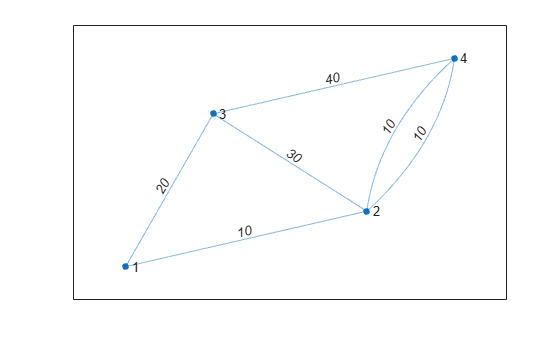# findedge

## 语法

``[sOut,tOut] = findedge(G)``
``[sOut,tOut] = findedge(G,idx)``
``idxOut = findedge(G,s,t)``
``[idxOut,m] = findedge(G,s,t)``

## 说明

`对于图 `G` 中的所有边，`[sOut,tOut] = findedge(G)` 返回的源和目标节点 ID `sOut` 和 `tOut`。`

``[sOut,tOut] = findedge(G,idx)` 查找 `idx` 指定的边的源和目标节点。`

`对于源和目标节点对组 `s` 和 `t` 指定的边，`idxOut = findedge(G,s,t)` 返回数值边索引 `idxOut`。边索引对应于图的 `G.Edges` 表中的行 `G.Edges.Edge(idxOut,:)`。如果 `s` 和 `t` 之间存在多条边，则返回它们的所有索引。边索引 `0` 表示边不在图中。`
``[idxOut,m] = findedge(G,s,t)` 还返回向量 `m`，指示哪个节点对 `(s,t)` 与 `idxOut` 中的每个边索引关联。这在相同两个节点之间存在多条边时很有用。`

## 示例

```s = [1 1 2 2 2 3 3 3]; t = [2 3 3 4 5 6 7 5]; G = graph(s,t)```
```G = graph with properties: Edges: [8x1 table] Nodes: [7x0 table] ```
`idxOut = findedge(G,[1 3],[2 5])`
```idxOut = 2×1 1 6 ```

`idxOut` 包含每条指定边的 `G.Edges.EndNodes` 的行索引。

```s = {'a' 'a' 'b' 'b' 'c' 'c'}; t = {'b' 'c' 'd' 'e' 'f' 'g'}; G = graph(s,t); G.Edges```
```ans=6×1 table EndNodes ______________ {'a'} {'b'} {'a'} {'c'} {'b'} {'d'} {'b'} {'e'} {'c'} {'f'} {'c'} {'g'} ```
`[sOut,tOut] = findedge(G)`
```sOut = 6×1 1 1 2 2 3 3 ```
```tOut = 6×1 2 3 4 5 6 7 ```

```s = [1 1 1 1 2 2 3 3 4 4]; t = [2 3 4 5 6 7 8 9 10 11]; G = digraph(s,t)```
```G = digraph with properties: Edges: [10x1 table] Nodes: [11x0 table] ```
`[sOut,tOut] = findedge(G,[3 7])`
```sOut = 2×1 1 3 ```
```tOut = 2×1 4 8 ```

```s = [1 1 2 3]; t = [2 3 3 4]; weights = [10 20 30 40]; G = graph(s,t,weights)```
```G = graph with properties: Edges: [4x2 table] Nodes: [4x0 table] ```

`G.Edges.Weight(findedge(G,1,3))`
```ans = 20 ```

```s = [1 1 2 3 2 2]; t = [2 3 3 4 4 4]; weights = [10 20 30 40 10 10]; G = graph(s,t,weights); plot(G,'EdgeLabel',G.Edges.Weight)``````s = [3 2]; t = [2 4]; w = [1 4]; [idxOut, m] = findedge(G, s, t)```
```idxOut = 3×1 3 4 5 ```
```m = 3×1 1 2 2 ```
```G.Edges.Weight(idxOut) = w(m); plot(G,'EdgeLabel',G.Edges.Weight)```## 输出参数

`idxOut` 的长度对应于输入中的节点对的数量，除非输入图是多重图。